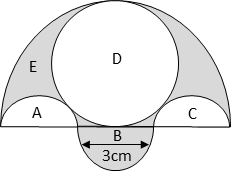# 2017 CBSE Board Solution

Class 10 Math - Set 3 - Q13. Mensuration

#### Video ExplanationArea of the shaded region = area of semicircle E of radius 4.5 cm – (area of circle D of diameter 4.5cm + area of semicircle A of diameter 3cm)

Semicircles B and C cancel out each other. So there is no need to add B and subtract C.

Diameter of circle D = 4.5 = $$frac{9}{2}$. Therefore, radius = $\frac{9}{4}$ Diameter of semicircle A = 3 = Therefore, radius = $\frac{3}{2}$ Area of semicircle E of radius $\frac{9}{2}$ = $\frac {{{\pi }r}^{2}} {2}$ = $\frac {\pi } {2}$ $$$frac {9} {2}{$}^{2})= $\frac{81\pi}{8}$ Area of circle D of radius $\frac{9}{4}$ =$πr2) = π$frac {9} {4}{)}^{2}) = $\frac{81π}{16}$ Area of semicircle A of radius $\frac{3}{2}$ = $\frac {{{\pi }r}^{2}} {2}$ = $\frac {\pi } {2}$ $$\frac {3} {2}{$}^{2}) = $\frac{9\pi}{8}$

Area of shaded region = $\frac{81\pi}{8}$ - $\frac{81\pi}{16}$ - $\frac{9\pi}{8}$
= $\frac{162\pi - 81\pi - 18\pi}{16}$ = $\frac{63\pi}{16}$

Take π = $\frac{22}{7}$

Therefore, $\frac{63\pi}{16}$ = $\frac{63}{16}$ × $\frac{22}{7}$ = $\frac{9 * 11}{8}$ = $\frac{99}{8}$ = 12.375 cm2

.